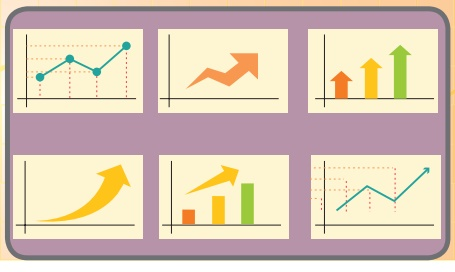Home | | Statistics 12th Std | Introduction

# Introduction

The correlation coefficient is an useful statistical tool for describing the type ( positive or negative or uncorrelated ) and intensity of linear relationship (such as moderately or highly) between two variables.

REGRESSION ANALYSIS

Francis Galton (1822 -1911) was born in a wealthy family. The youngest of nine children, he appeared as an intelligent child. Galton’s progress in education was not smooth. He dabbled in medicine and then studied Mathematics at Cambridge. In fact he subsequently freely acknowledged his weakness in formal Mathematics, but this weakness was compensated by an exceptional ability to understand the meaning of data. Many statistical terms, which are in current usage were coined by Galton. For example, correlation is due to him, as is regression, and he was the originator of terms and concepts such as quartile, decile and percentile, and of the use of median as the midpoint of a distribution.The concept of regression comes from genetics and was popularized by Sir Francis Galton during the late 19th century with the publication of regression towards mediocrity in hereditary stature. Galton observed that extreme characteristics (e.g., height) in parents are not passed on completely to their offspring. An examination of publications of Sir Francis Galton and Karl Pearson revealed that Galton's work on inherited characteristics of sweet peas led to the initial conceptualization of linear regression. Subsequent efforts by Galton and Pearson brought many techniques of multiple regression and the product-moment correlation coefficient.## LEARNING OBJECTIVES

The student will be able to

·              know the concept of regression, its types and their uses.

·              fit best line of regression by applying the method of least squares.

·              calculate the regression coefficient and interpret the same.

·              know the uses of regression coefficients.

·              distinguish between correlation analysis and regression analysis.

## Introduction

The correlation coefficient is an useful statistical tool for describing the type ( positive or negative or uncorrelated ) and intensity of linear relationship (such as moderately or highly) between two variables. But it fails to give a mathematical functional relationship for prediction purposes. Regression analysis is a vital statistical method for obtaining functional relationship between a dependent variable and one or more independent variables. More specifically, regression analysis helps one to understand how the typical value of the dependent variable (or ‘response variable’) changes when any one of the independent variables (regressor(s) or predictor(s)) is varied, while the other independent variables are held fixed. It helps to determine the impact of changes in the value(s) of the the independent variable(s) upon changes in the value of the dependent variable. Regression analysis is widely used for prediction.

Tags : Regression Analysis , 12th Statistics : Chapter 5 : Regression Analysis
Study Material, Lecturing Notes, Assignment, Reference, Wiki description explanation, brief detail
12th Statistics : Chapter 5 : Regression Analysis : Introduction | Regression Analysis